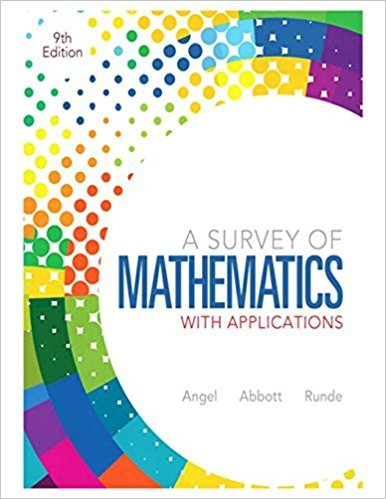×
×

# Solutions for Chapter 9.7: Geometry## Full solutions for A Survey of Mathematics with Applications | 9th Edition

ISBN: 9780321759665Solutions for Chapter 9.7: Geometry

Solutions for Chapter 9.7
4 5 0 297 Reviews
12
1
##### ISBN: 9780321759665

This textbook survival guide was created for the textbook: A Survey of Mathematics with Applications, edition: 9. This expansive textbook survival guide covers the following chapters and their solutions. A Survey of Mathematics with Applications was written by and is associated to the ISBN: 9780321759665. Chapter 9.7: Geometry includes 27 full step-by-step solutions. Since 27 problems in chapter 9.7: Geometry have been answered, more than 70082 students have viewed full step-by-step solutions from this chapter.

Key Math Terms and definitions covered in this textbook
• Associative Law (AB)C = A(BC).

Parentheses can be removed to leave ABC.

• Column picture of Ax = b.

The vector b becomes a combination of the columns of A. The system is solvable only when b is in the column space C (A).

• Commuting matrices AB = BA.

If diagonalizable, they share n eigenvectors.

• Dimension of vector space

dim(V) = number of vectors in any basis for V.

• Distributive Law

A(B + C) = AB + AC. Add then multiply, or mUltiply then add.

• Fourier matrix F.

Entries Fjk = e21Cijk/n give orthogonal columns FT F = nI. Then y = Fe is the (inverse) Discrete Fourier Transform Y j = L cke21Cijk/n.

• Full column rank r = n.

Independent columns, N(A) = {O}, no free variables.

• Fundamental Theorem.

The nullspace N (A) and row space C (AT) are orthogonal complements in Rn(perpendicular from Ax = 0 with dimensions rand n - r). Applied to AT, the column space C(A) is the orthogonal complement of N(AT) in Rm.

• Hypercube matrix pl.

Row n + 1 counts corners, edges, faces, ... of a cube in Rn.

• Incidence matrix of a directed graph.

The m by n edge-node incidence matrix has a row for each edge (node i to node j), with entries -1 and 1 in columns i and j .

• Inverse matrix A-I.

Square matrix with A-I A = I and AA-l = I. No inverse if det A = 0 and rank(A) < n and Ax = 0 for a nonzero vector x. The inverses of AB and AT are B-1 A-I and (A-I)T. Cofactor formula (A-l)ij = Cji! detA.

• Left nullspace N (AT).

Nullspace of AT = "left nullspace" of A because y T A = OT.

• Linear combination cv + d w or L C jV j.

• Lucas numbers

Ln = 2,J, 3, 4, ... satisfy Ln = L n- l +Ln- 2 = A1 +A~, with AI, A2 = (1 ± -/5)/2 from the Fibonacci matrix U~]' Compare Lo = 2 with Fo = O.

• Minimal polynomial of A.

The lowest degree polynomial with meA) = zero matrix. This is peA) = det(A - AI) if no eigenvalues are repeated; always meA) divides peA).

• Permutation matrix P.

There are n! orders of 1, ... , n. The n! P 's have the rows of I in those orders. P A puts the rows of A in the same order. P is even or odd (det P = 1 or -1) based on the number of row exchanges to reach I.

• Schur complement S, D - C A -} B.

Appears in block elimination on [~ g ].

• Spectrum of A = the set of eigenvalues {A I, ... , An}.

Spectral radius = max of IAi I.

• Unitary matrix UH = U T = U-I.

Orthonormal columns (complex analog of Q).

• Volume of box.

The rows (or the columns) of A generate a box with volume I det(A) I.

×